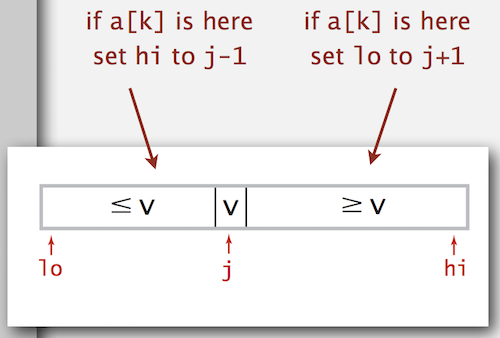Nothing special here. It’s just a blog post for summarising my algorithm learning course. Although this was already taught in the University, I didn’t even know that it can be used for Selection Problem

# Selection Problem

Given an array of N items, find a kth smallest item. For example, an array A = [5, 2, 9, 4, 10, 7], the 3rd smallest item is 5, the 2nd smallest item is 4 and the smallest item is 2

# The idea

• Based on Quick Sort
• Partition the array so that
• Entry a[j] is in place.
• No larger entry to the left of j
• No smaller entry to the right of j
• Repeat in one sub-array, depending on j, finished when j equals k

# Java Implementation

public static Comparable select(Comparable[] a, int k) {
StdRandom.shuffle(a);
int lo = 0, hi = a.length - 1;
while (hi > lo)
{
int j = partition(a, lo, hi);
if (j < k) lo = j + 1;
else if (j > k) hi = j - 1;
else return a[k];
}
return a[k];
}


The idea is that, after each partition, the item a[j] will be moved to the right place. So it will become the jth smallest item. Repeat that process until we can find j = k.# Performance

Quick-select takes linear time on average. Quick-select uses ~½ N2 compares in the worst case, but (as with quicksort) the random shuffle provides a probabilistic guarantee.